Courses

# NEET Previous Year Questions (2014-20): Mechanical Properties of Fluids Class 11 Notes | EduRev

## Class 11 : NEET Previous Year Questions (2014-20): Mechanical Properties of Fluids Class 11 Notes | EduRev

The document NEET Previous Year Questions (2014-20): Mechanical Properties of Fluids Class 11 Notes | EduRev is a part of the Class 11 Course Physics 31 Years NEET Chapterwise Solved Papers.
All you need of Class 11 at this link: Class 11

Q 1. A soap bubble, having radius of 1 mm, is blown from a detergent solution having a surface tension of 2.5 × 10-2 N/m. The pressure inside the bubble equals at a point Z0 below the free surface of water in a container. Taking g = 10 m/s2, density of water = 103 kg/m3, the value of Z0 is     
A: 100 cm
B: 10 cm
C: 1 cm
D: 0.5 cm
Ans:
C
Solution:
Excess pressure = 4T/R, Gauge pressure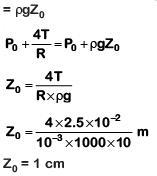Q 2. A small hole with area area of cross-section 2 mm2 is present near the bottom of a fully filled open tank of height 2 m. Taking g = 10 m/s2, the rate of flow of water through the open hole would be nearly    
A: 12.6 × 10–6 m3/s
B: 8.9 × 10–6 m3/s
C: 2.23 × 10–6 m3/s
D: 6.4 × 10–6 m3/s
Ans:
A
Solution: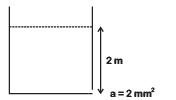Rate of liquid flow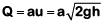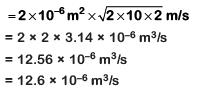Q 3. A small sphere of radius 'r' falls from rest in a viscous liquid. As a result, heat is produced due to viscous force. The rate of production of heat when the sphere attains its terminal velocity, is proportional to:-    
A: r3
B: r2
C: r5
D: r4
Ans:
C
Solution: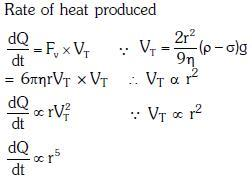Q 4. A U tube with both ends open to the atmosphere, is partially filled with water. Oil, which is immiscible with water, is poured into one side until it stands at a distance of 10 mm above the water level on the other side. Meanwhile the water rises by 65 mm from its original level (see diagram). The density of the oil is:-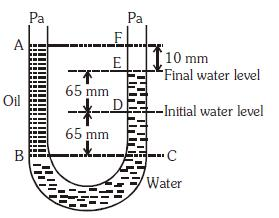A: 425 kg m–3
B: 800 kg m–3
C: 928 kg m–3
D: 650 kg m–3
Ans:
C
Solution: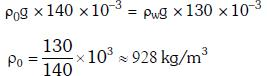Q 5. Two non-mixing liquids of densities ρ and nρ (n > 1) are put in container. The height of each liquid is h. A solid cylinder of length L and density d is put in this container. The cylinder floats with its axis vertical and length pL (p < 1) in the denser liquid. The density d is equal to    
A: {1 + (n - 1)p}ρ
B: {1 + (n + 1)p}ρ
C: {2+(n + 1)p}ρ
D: {2 + (n - 1)p}ρ
Ans:
A
Solution: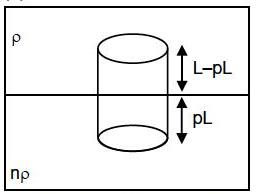wt of body = upthrust by the two liquids
If A = Area of section then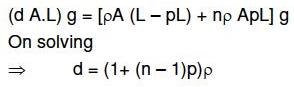Q 6. The approximate depth of an ocean is 2700 m. The compressibility of water is 45.4 x 10-11 Pa-1 and density of water is 103 kg/m3. What fractional compression of water will be obtained at the bottom of the ocean?    
A: 1.4 x 10-2
B: 0.8 x 10-2
C: 1.0 x 10-2
D: 1.2 x 10-2
Ans:
D
Solution: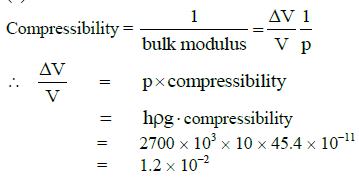Q 7. A wind with speed 40 m/s blows parallel to the roof of a house. The area of the roof is 250 m2. Assuming that the pressure inside the house is atmospheric pressure, the force exerted by the wind on the roof and the direction of the force will be:
(Pair = 1.2 kg/m3)    
A: 2.4 x 105 N, downwards
B: 4.8 x 105 N, downwards
C: 4.8 x 105 N,upwards
D: 2.4 x 105 N, upwards
Ans:
D
Solution: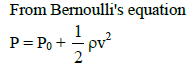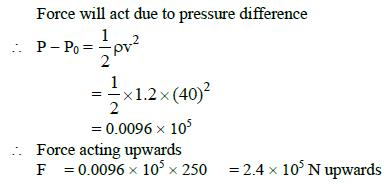Q 8. A certain number of spherical drops of a liquid of radius ‘r’ coalesce to form a single drop of radius ‘R’ and volume ‘V’. If ‘T’ is the surface tension of the liquid, then:    
A: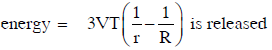B: energy is neither released nor absorbed
C: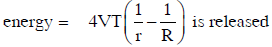D: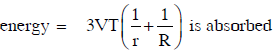Ans:
A
Solution: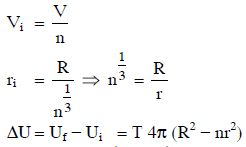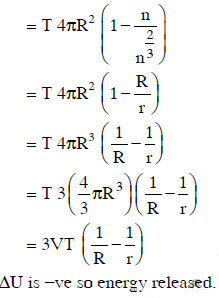Offer running on EduRev: Apply code STAYHOME200 to get INR 200 off on our premium plan EduRev Infinity!

28 docs|50 tests

,

,

,

,

,

,

,

,

,

,

,

,

,

,

,

,

,

,

,

,

,

;